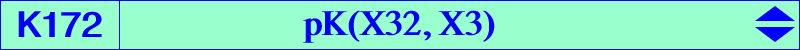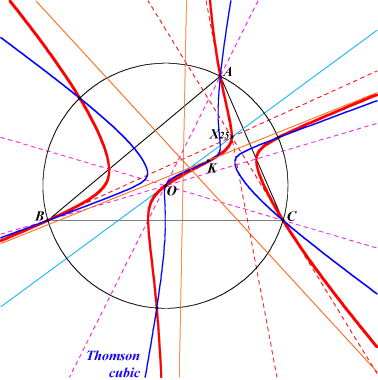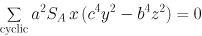X(3), X(6), X(25), X(55), X(56), X(64), X(154), X(198), X(1033), X(1035), X(1436) vertices of the tangential triangle vertices of the Thomson triangle vertices of the tangential triangle of the Thomson triangle, see K1109 and also Table 27, paragraph Lemoine point of T(P) isogonal conjugates of the CPCC or H-cevian points, see Table 11K346 is the locus of the poles of pKs meeting the circumcircle at the same points as the Thomson cubic K002. This is the case of K167 = pK(X184, X6) and K172 = pK(X32, X3). The locus of the pivots of such cubics is K002 itself and the locus of the isopivots is K172. K172 is the isogonal transform of the Lucas cubic K007. See generalizations at CL023 and CL060. See also Table 34. The inversive image of K172 in the circumcircle is Q071. K172 is anharmonically equivalent to the Thomson cubic. See Table 21. Let ABC be a triangle, P a point, and PaPbPc the pedal triangle of P. La, Lb, Lc are the parallels to BC, CA, AB, resp., through P. Denote by : Lab = La /\ AB, Lac = La /\ AC, Ab = the orthogonal projection of Lab on Lc, Ac = the orthogonal projection of Lac on Lb, A' = BAb /\ CAc, and similarly define B', C'. The locus of P such that ABC, A'B'C' are perspective is the Thomson cubic. The locus of the perspector is K172. Note that the cubic is also a pK with pivot K, invariant under the isoconjugation with respect to the tangential triangle which swaps K and O. (Antreas Hatzipolakis, Jean-Pierre Ehrmann, Hyacinthos #8480-83-87) The asymptotic directions of K172 are those of pK(X6, X2979) where X2979 = a^2(b^4 + c^4 + b^2c^2 - a^2b^2 - a^2c^2) : : , intersection of the lines X(3)X(54) and X(2)X(51). K172 is a member of the pencil generated by the Thomson cubic K002 and the union of the circumcircle and the Brocard axis. This also contains K167, K297. K172 and K1114 generate another pencil of cubics studied in Table 71. We met K172 in the solution of the Darboux problem : one considers the conics circumscribed to a triangle such that the normals at the three vertices of the triangle are concurrent at a point P. One asks the locus of the foot of the fourth normal passing through P (literal translation). In , it is shown that the locus is a 7th degree curve which is the isogonal transform of the inverse (in the circumcircle) of a non-identified cubic. It appears that this cubic is K172 and consequently the locus is the antigonal image of the Lucas cubic (see Hyacinthos #7825 & sq.). Antigonal is defined here. We call this locus the Darboux septic or Q001.  André Haarbleicher: De l'emploi des droites isotropes comme axes de coordonnées. Nouvelle géométrie du triangle. Paris, Gauthier-Villars, 1931, pp. 61-70. See also Hyacinthos #8509-11-12-15. *** K576 is the locus of the pseudo-poles of central cubics psK with center O such as K004, K426, K443. The loci of the pseudo-pivots and the pseudo-isopivots are K099 and K172 respectively.